Published © GPL3+

# Arduino Negative Voltmeter

3-channel voltmeter with the ability to measure both negative & positive voltages on the same circuit or 3 different circuits simultaneously

BeginnerFull instructions provided4 hours2,038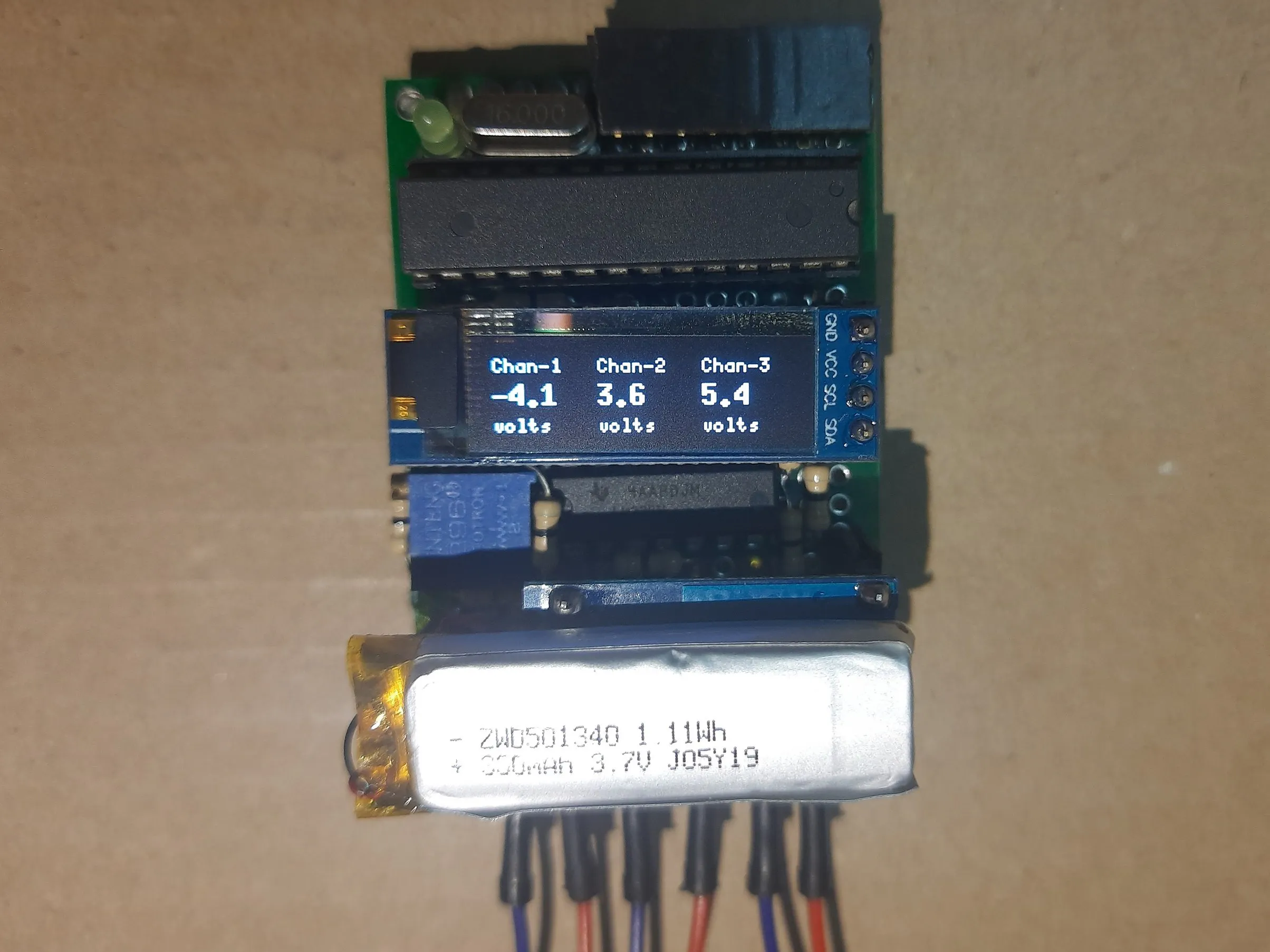## Things used in this project

### Hardware components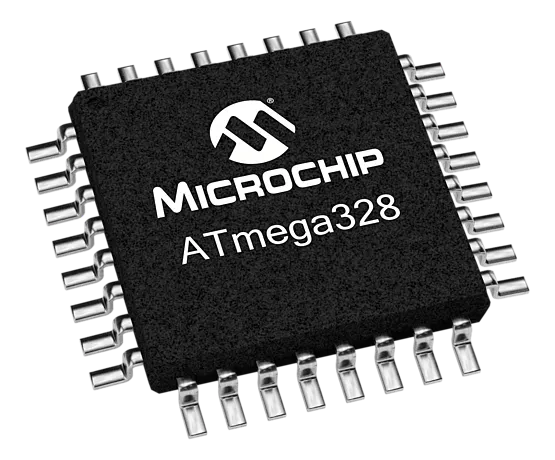Microchip Technology ATmega328
×1Arduino UNO
×1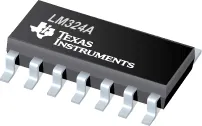Texas Instruments General Purpose Quad Op-Amp
×1
 I2C OLED 128x32
×1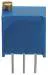Multi-Turn Precision Potentiometer- 10k ohms (25 Turn)
×1
 TP4056 LiPo charger
×1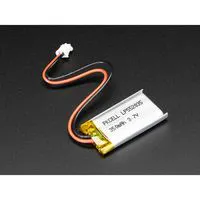Battery, 3.7 V
×1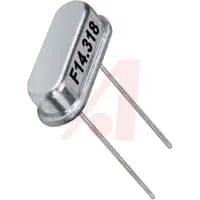16 MHz Crystal
×1
 FR4 proto board 4x6
×1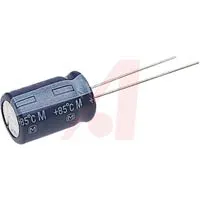Capacitor 10 µF
×1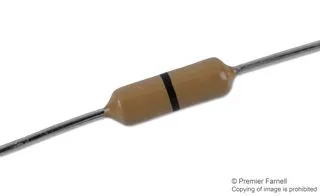Through Hole Resistor, 0 ohm
×30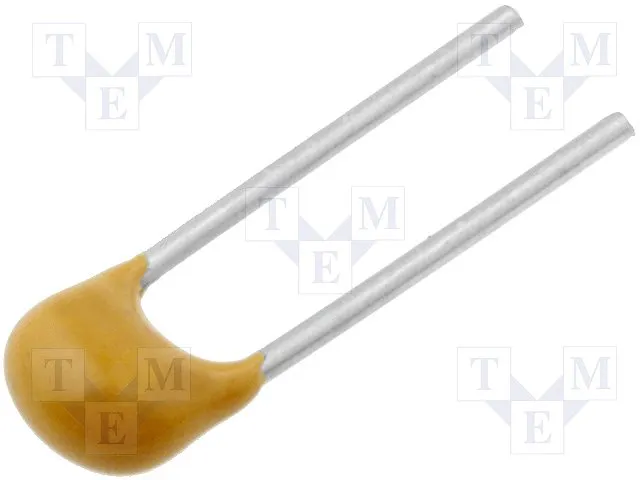Capacitor 100 nF
×1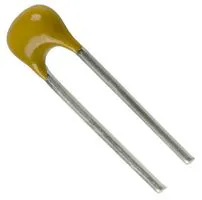Capacitor 22 pF
×2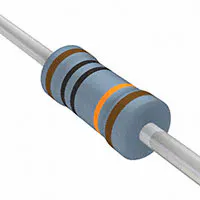Resistor 100k ohm
×3
 Resistor 10M Ohm
×6

### Software apps and online servicesArduino IDE

### Hand tools and fabrication machinesSoldering iron (generic)

Read more

## Schematics

### Schematic

ver 1.01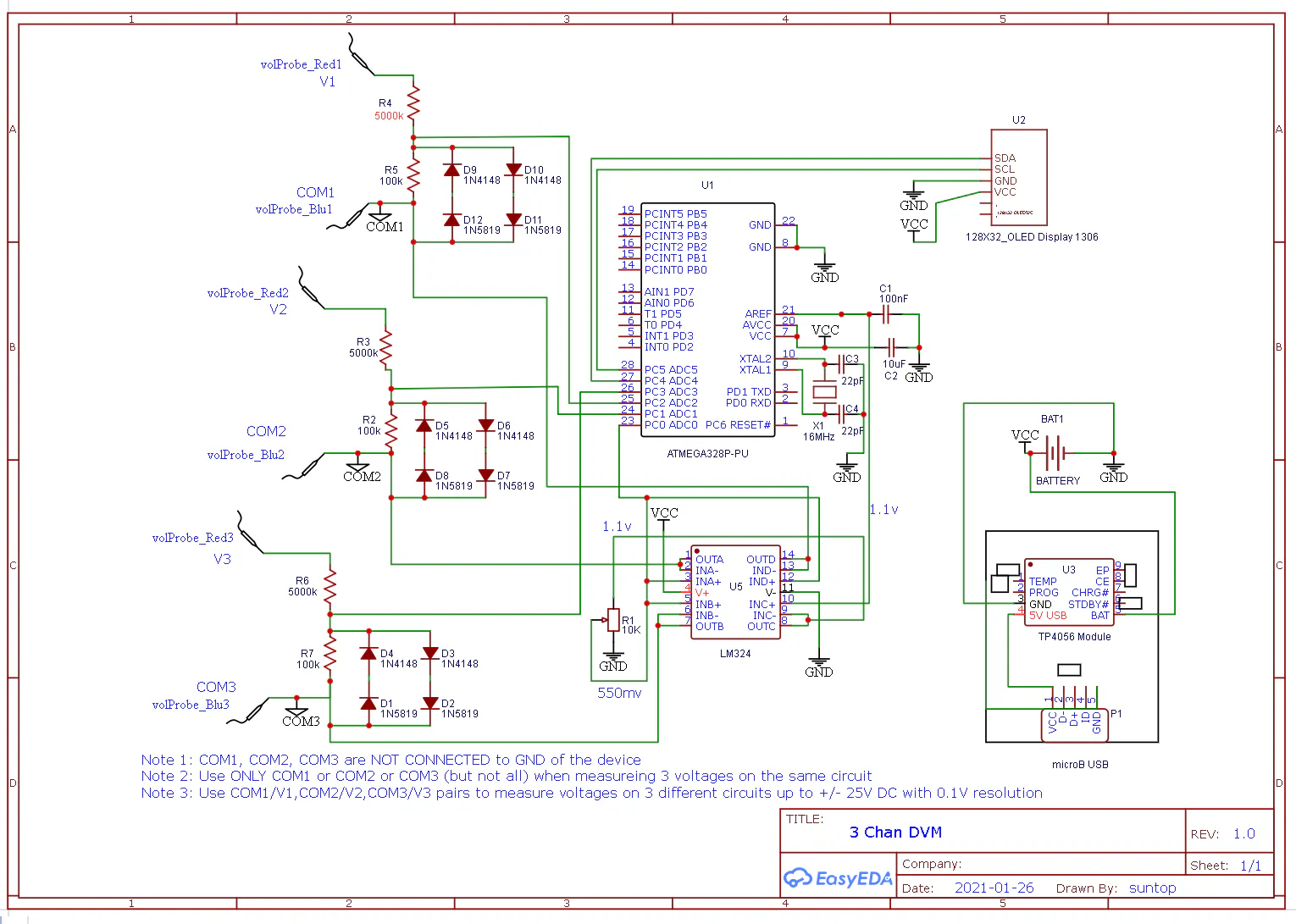## Code

### main

C/C++
```// i2c and u8g library for 1306 oled display

#include <Wire.h>
#include "U8glib.h"

#define R_LOW 100           // 100k or 10k resistor, 1%, smd1206, 1/10 watt
#define R_HIGH 5000         // 5M or 500k  resistor, 1%, smd1206, 1/10 watt
#define AREF 1.1            // Aref pin voltage
#define ADC_RESOLUTION 1023 // 10 bit ADC

U8GLIB_SSD1306_128X32 u8g(U8G_I2C_OPT_NONE);     // I2C OLED  Display instance
long half_Aref = 0; // adc 0 for reading half AREF = 550mv, should read 512
long ch_1 = 0;      // adc 1 for reading voltage divided ratio of external V1
long ch_2 = 0;      // adc 2 for reading voltage divided ratio of external V2
long ch_3 = 0;      // adc 3 for reading voltage divided ratio of external V3
float V1 = 0.0;     // calculating measured V1 from ADC ch 1
float V2 = 0.0;     // calculating measured V1 from ADC ch 2
float V3 = 0.0;     // calculating measured V1 from ADC ch 3
int dump = 0;       // discarding first adc conversion

/// Oled Loop Draw Function ///
void draw(void)
{

// set font for text
u8g.setFont(u8g_font_5x8);
u8g.drawStr( 0, 6, "Chan-1   Chan-2   Chan-3");
// set font for number
u8g.setFont(u8g_font_7x13B);
// pring voltages measured on OLED
u8g.setPrintPos(0, 20);u8g.print(V1,1);
u8g.setPrintPos(45, 20);u8g.print(V2,1);
u8g.setPrintPos(90, 20);u8g.print(V3,1);
// set font and print units
u8g.setFont(u8g_font_5x8);
u8g.drawStr( 0, 32, "volts    volts    volts");

}
//////////////////////////////////////////////////////////

/// void setup ///

void setup(void)
{

delay(500);                // delay for idk
analogReference(INTERNAL); // set 1.1v internal reference
delay(500);                // delay to stabilize aref voltage
u8g.setRot180();           // change display orientation

}

//////////////////////////////////////////////////////////

/// void loop ///

void loop(void)
{

delay(5); // delay before using I2C OLED
// refresh i2c oled with updated value
u8g.firstPage();
do {
draw();
} while( u8g.nextPage() );

delay(5);

// clear variables old value
half_Aref = 0;
ch_1 = 0;
ch_2 = 0;
ch_3 = 0;

/// Half AREF measurement ///

dump = analogRead(A0); // discard first adc convertion
delay(10); // delay for channel switching stabilizaion

// take 150 samples and add them up
for (int i=0; i<150;i++)
{
half_Aref = half_Aref+analogRead(A0);
//    delay(2);
}

// average 150 samples for improved accuracy
half_Aref = half_Aref/150;

//////////////////////////////////////////////////////////////////////

/// ADC Chan1 measurement for voltage V1 ///

dump = analogRead(A1); // discard first adc convertion
delay(10); // delay for channel switching stabilizaion

// take 150 samples and add them up
for (int i=0; i<150;i++)
{
ch_1 = ch_1+analogRead(A1);
//    delay(2);
}

ch_1 = ch_1/150;        // average 150 samples for improved accuracy
ch_1 = ch_1- half_Aref; // calculate adc differential for measured V1
V1 = ch_1*((R_LOW+R_HIGH)/R_LOW)*(AREF)/ADC_RESOLUTION; // calc V1
///////////////////////////////////////////////////////////////////////

/// ADC Chan2 measurement for voltage V2 ///

dump = analogRead(A2); // discard first adc convertion
delay(10); // delay for channel switching stabilizaion

// take 150 samples and add them up
for (int i=0; i<150;i++)
{
ch_2 = ch_2+analogRead(A2);
//    delay(2);
}

ch_2 = ch_2/150;        // average 150 samples for improved accuracy
ch_2 = ch_2- half_Aref; // calculate adc differential for measured V2
V2 = ch_2*((R_LOW+R_HIGH)/R_LOW)*(AREF)/ADC_RESOLUTION; // calc V2
///////////////////////////////////////////////////////////////////////

/// ADC Chan3 measurement for voltage V3 ///

dump = analogRead(A3); // discard first adc convertion
delay(10); // delay for channel switching stabilizaion

// take 150 samples and add them up
for (int i=0; i<150;i++)
{
ch_3 = ch_3+analogRead(A3);
//    delay(2);
}

ch_3 = ch_3/150;        // average 150 samples for improved accuracy
ch_3 = ch_3- half_Aref; // calculate adc differential for measured V3
V3 = ch_3*((R_LOW+R_HIGH)/R_LOW)*(AREF)/ADC_RESOLUTION; // calc V3
///////////////////////////////////////////////////////////////////////

}

/// Void Loop ends here ///
///////////////////////////////////////////////////////////////////////
```

## Credits

### Shahariar

60 projects • 209 followers
"What Kills a 'Great life' is a 'Good Life', which is Living a Life Inside While Loop"